# Generalised Linear Models with brms

This tutorial provides an introduction to Bayesian GLM (genearlised linear models) with non-informative priors using the brms package in R. If you have not followed the Intro to Frequentist (Multilevel) Generalised Linear Models (GLM) in R with glm and lme4 tutorial, we highly recommend that you do so, because it offers more extensive information about GLM. If you are not familar with Bayesian inference, we also recommend that you read this tutorial Building a Multilevel Model in BRMS Tutorial: Popularity Data prior to using this tutorial.

The current tutorial specifically focuses on the use of Bayesian logistic regression in both binary-outcome and count/porportion-outcome scenarios, and the respective approaches to model evaluation. The tutorial uses the Thai Educational Data example in Chapter 6 of the book Multilevel analysis: Techniques and applications. Furthermore, the tutorial briefly demonstrates the multilevel extension of Bayesian GLM models.

This tutorial follows this structure:
1. Preparation;
2. Introduction to GLM;
3. Thai Educational Data;
4. Data Preparation;
5. Bayesian Binary (Bernoulli) Logistic Regression;
6. Bayesian Binomial Logistic Regression;
7. Bayesian Multilevel Logistic Regression.

Note that this tutorial is meant for beginners and therefore does not delve into technical details and complex models. For a detailed introduction into frequentist multilevel models, see this LME4 Tutorial. For an extensive overview of GLM models, see here. If you want to use the Bayesian approach for your own research, we recommend that you follow the WAMBS-checklist.

## 1. Preparation

This tutorial expects:
– Installation of R packages brms for Bayesian (multilevel) generalised linear models (this tutorial uses version 2.9.0). Because of some special dependencies, for brms to work, you still need to install a couple of other things. See this tutorial on how to install brms. Note that currently brms only works with R 3.5.3 or an earlier version;
– Installation of R package tidyverse for data manipulation and plotting with ggplot2;
– Installation of R package haven for reading sav format data;
– Installation of R package ROCR for calculating area under the curve (AUC);
– Installation of R package sjstats for calculating intra-class correlation (ICC). Remember to install version 0.17.5 (using the command install_version("sjstats", version = "0.17.5") after loading the package devtools, because the latest version of sjstats does not support the ICC function anymore);
– Installation of R package modelr for data manipulation;
– Installation of R package tidybayes for extraction, manipulation, and visualisation of posterior draws from Bayesian models;
– Basic knowledge of hypothesis testing and statistical inference;
– Basic knowledge of Bayesian statistical inference;
– Basic knowledge of coding in R;
– Basic knowledge of plotting and data manipulation with tidyverse.

## 2. Introduction to Genearlised Linear Models (GLM)

If you are already familar with generalised linear models (GLM), you can proceed to the next section. Otherwise, click “Read More” to learn about GLM.

Recall that in a linear regression model, the object is to model the expected value of a continuous variable, $$Y$$, as a linear function of the predictor, $$\eta = X\beta$$. The model structure is thus: $$E(Y) = X\beta + e$$, where $$e$$ refers to the residual error term. The linear regression model assumes that $$Y$$ is continous and comes from a normal distribution, that $$e$$ is normally distributed and that the relationship between the linear predictor $$\eta$$ and the expected outcome $$E(Y)$$ is strictly linear. However, these assumptions are easily violated in many real world data examples, such as those with binary or proportional outcome variables and those with non-linear relationships between the predictors and the outcome variable. In these scenarios where linear regression models are clearly inappropriate, generalised linear models (GLM) are needed.

The GLM is the genearlised version of linear regression that allows for deviations from the assumptions underlying linear regression. The GLM generalises linear regression by assuming the dependent variable $$Y$$ to be generated from any particular distribution in an exponential family (a large class of probability distributions that includes the normal, binomial, Poisson and gamma distributions, among others). In this way, the distribution of $$Y$$ does not necessarily have to be normal. In addition, the GLM allows the linear predictor $$\eta$$ to be connected to the expected value of the outcome variable, $$E(Y)$$, via a link function $$g(.)$$. The outcome variable, $$Y$$, therefore, depends on $$\eta$$ through $$E(Y) = g^{-1}(\eta) = g^{-1}(X\beta)$$. In this way, the model does not assume a linear relationship between $$E(Y)$$ and $$\eta$$; instead, the model assumes a linear relationship between $$E(Y)$$ and the transformed $$g^{-1}(\eta)$$.

This tutorial focuses on the Bayesian version of the probably most popular example of GLM: logistic regression. Logistic regression has two variants, the well-known binary logistic regression that is used to model binary outcomes (1 or 0; “yes” or “no”), and the less-known binomial logistic regression suited to model count/proportion data.

Binary logistic regression assumes that $$Y$$ comes from a Bernoulli distribution, where $$Y$$ only takes a value of 1 (target event) or 0 (non-target event). Binary logistic regression connects $$E(Y)$$ and $$\eta$$ via the logit link $$\eta = logit(\pi) = log(\pi/(1-\pi))$$, where $$\pi$$ refers to the probability of the target event ($$Y = 1$$).

Binomial logistic regression, in contrast, assumes a binomial distribution underlying $$Y$$, where $$Y$$ is interpreted as the number of target events, can take on any non-negative integer value and is binomially distributed with regards to $$n$$ number of trials and $$\pi$$ probability of the target event. The link function is the same as that of binary logistic regression.

The next section details the exampler data (Thai Educational Data) in this tutorial, followed by the demonstration of the use of Bayesian binary, Bayesian binomial logistic regression and Bayesian multilevel binary logistic regression. For the frequentist versions of these models, see the Intro to Frequentist (Multilevel) Generalised Linear Models (GLM) in R with glm and lme4 tutorial.

## 3. Thai Educational Data

The data used in this tutorial is the Thai Eduational Data that is also used as an example in Chapter 6 of Multilevel analysis: Techniques and applications. The data can be downloaded from here.

The data stems from a national survey of primary education in Thailand (Raudenbush & Bhumirat, 1992). Each row in the data refers to a pupil. The outcome variable REPEAT is a dichotomous variable indicating whether a pupil has repeated a grade during primary education. The SCHOOLID variable indicates the school of a pupil. The person-level predictors include: SEX (0 = female, 1 = male) and PPED (having had preschool education, 0 = no, 1 = yes). The school-level is MSESC, representing school mean SES (socio-economic status) scores.

The main research questions that this tutorial seeks to answer using the Thai Educational Data are:

1. Ignoring the clustering structure of the data, what are the effects of gender and preschool education on whether a pupil repeats a grade?
2. Ignoring the clustering structure of the data, what is the effect of school mean SES on the proportion of pupil repeating a grade?
3. Considering the clustering structure of the data, what are the effects of gender, preschool education and school mean SES on whether a pupil repeats a grade?

These three questions are answered by using these following models, respectively: Bayesian binary logistic regressioin; Bayesian binomial logistic regression; Bayesian multilevel binary logistic regression.

## 4. Data Preparation

# if you dont have these packages installed yet, please use the install.packages("package_name") command.
library(tidyverse) # for data manipulation and plots
library(sjstats) #for calculating intra-class correlation (ICC)
library(ROCR) #for calculating area under the curve (AUC) statistics
library(brms) #for Bayesian (multilevel) generalised linear modelling
library(modelr) #for data manipulation
library(tidybayes) #for analysis of posterior draws of a Bayesian model

#### 4.2. Import Data

ThaiEdu_Raw <- read_sav("https://github.com/MultiLevelAnalysis/Datasets-third-edition-Multilevel-book/blob/master/chapter%206/Thaieduc/thaieduc.sav?raw=true")
head(ThaiEdu_Raw)
## # A tibble: 6 x 5
##   SCHOOLID       SEX      PPED    REPEAT MSESC
##      <dbl> <dbl+lbl> <dbl+lbl> <dbl+lbl> <dbl>
## 1    10101  0 [girl]   1 [yes]    0 [no]    NA
## 2    10101  0 [girl]   1 [yes]    0 [no]    NA
## 3    10101  0 [girl]   1 [yes]    0 [no]    NA
## 4    10101  0 [girl]   1 [yes]    0 [no]    NA
## 5    10101  0 [girl]   1 [yes]    0 [no]    NA
## 6    10101  0 [girl]   1 [yes]    0 [no]    NA

Alternatively, you can download the data directly from here and import it locally.

#### 4.3. Data Processing

ThaiEdu_New <- ThaiEdu_Raw %>%
mutate(SCHOOLID = factor(SCHOOLID),
SEX = if_else(SEX == 0, "girl", "boy"),
SEX = factor(SEX, levels = c("girl", "boy")),
PPED = if_else(PPED == 0, "no", "yes"),
PPED = factor(PPED, levels = c("no", "yes")))

head(ThaiEdu_New)
## # A tibble: 6 x 5
##   SCHOOLID SEX   PPED     REPEAT MSESC
##   <fct>    <fct> <fct> <dbl+lbl> <dbl>
## 1 10101    girl  yes      0 [no]    NA
## 2 10101    girl  yes      0 [no]    NA
## 3 10101    girl  yes      0 [no]    NA
## 4 10101    girl  yes      0 [no]    NA
## 5 10101    girl  yes      0 [no]    NA
## 6 10101    girl  yes      0 [no]    NA

#### 4.4. Inspect Missing Data

ThaiEdu_New %>%
summarise_each(list(~sum(is.na(.)))) %>%
gather()
## # A tibble: 5 x 2
##   key      value
##   <chr>    <int>
## 1 SCHOOLID     0
## 2 SEX          0
## 3 PPED         0
## 4 REPEAT       0
## 5 MSESC     1066

The data has 1066 observations missing for the MSESC variable. The treatment of missing data is a complicated topic on its own. For the sake of convenience, we simply list-wise delete the cases with missing data in this tutorial.

ThaiEdu_New <- ThaiEdu_New %>%
filter(!is.na(MSESC))

## 5. Bayesian Binary Logistic Regression (with Non-Informative Priors)

#### 5.1. Explore Data: number of REPEAT by SEX and PPED

ThaiEdu_New %>%
group_by(SEX) %>%
summarise(REPEAT = sum(REPEAT))
## # A tibble: 2 x 2
##   SEX   REPEAT
##   <fct>  <dbl>
## 1 girl     428
## 2 boy      639
ThaiEdu_New %>%
group_by(PPED) %>%
summarise(REPEAT = sum(REPEAT))
## # A tibble: 2 x 2
##   PPED  REPEAT
##   <fct>  <dbl>
## 1 no       673
## 2 yes      394

It seems that the number of pupils who repeated a grade differs quite a bit between the two genders, with more male pupils having to repeat a grade. More pupils who did not have preschool education repeated a grade. This observation suggests that SEX and PPED might be predictive of REPEAT.

#### 5.2. Fit a Bayesian Binary Logistic Regression Model

The brm function from the brms package performs Bayesian GLM. The brm has three basic arguments that are identical to those of the glm function: formula, family and data. However, note that in the family argument, we need to specify bernoulli (rather than binomial) for a binary logistic regression. The brm function has a few more additional (and necessary) arguments that glm does not offer: warmup specifies the burn-in period (i.e. number of iterations that should be discarded); iter specifies the total number of iterations (including the burn-in iterations); chains specifies the number of chains; inits specifies the starting values of the iterations (normally you can either use the maximum likelihood esimates of the parameters as starting values, or simply ask the algorithm to start with zeros); cores specifies the number of cores used for the algorithm; seed specifies the random seed, allowing for replication of results.

See below the specification of the binary logistic regression model with two predictors, without using informative priors.

Bayes_Model_Binary <- brm(formula = REPEAT ~ SEX + PPED,
data=ThaiEdu_New,
warmup = 500,
iter = 2000,
chains = 2,
inits= "0",
cores=2,
seed = 123)
## Compiling the C++ model
## Start sampling

#### 5.3. Model Convergence

Before looking at the model summary, we should check whether there is evidence of non-convergence for the two chains. To do so, we can use the stanplot function from the brms package.

First, we plot the caterpillar plot for each parameter of interest.

stanplot(Bayes_Model_Binary,
type = "trace")
## No divergences to plot.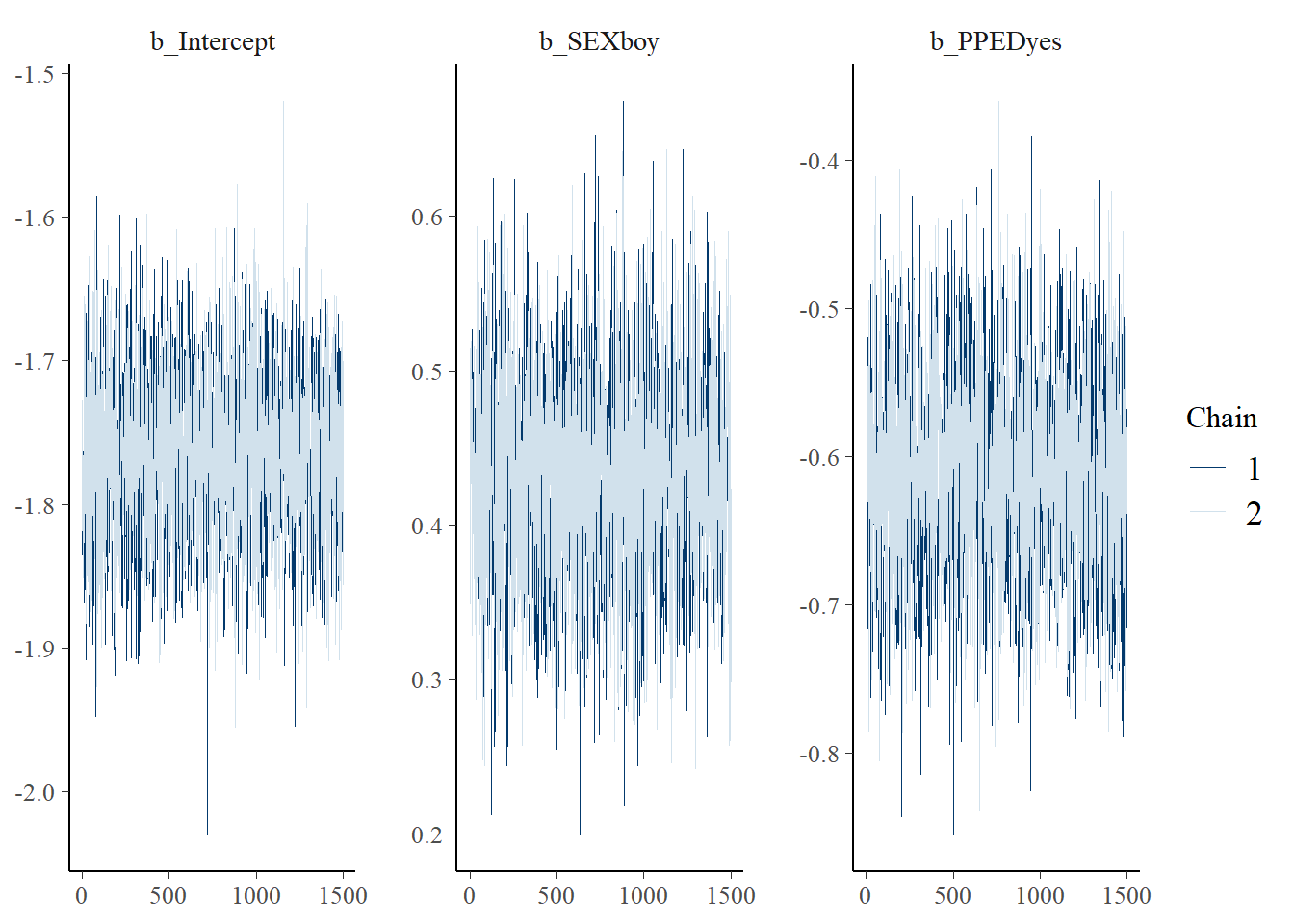The plot only shows the iterations after the burn-in period. The two chains mix well for all of the parameters and therefore, we can conclude no evidence of non-convergence.

We can also check autocorrelation, considering that the presence of strong autocorrelation would bias variance estimates.

stanplot(Bayes_Model_Binary,
type = "acf_bar")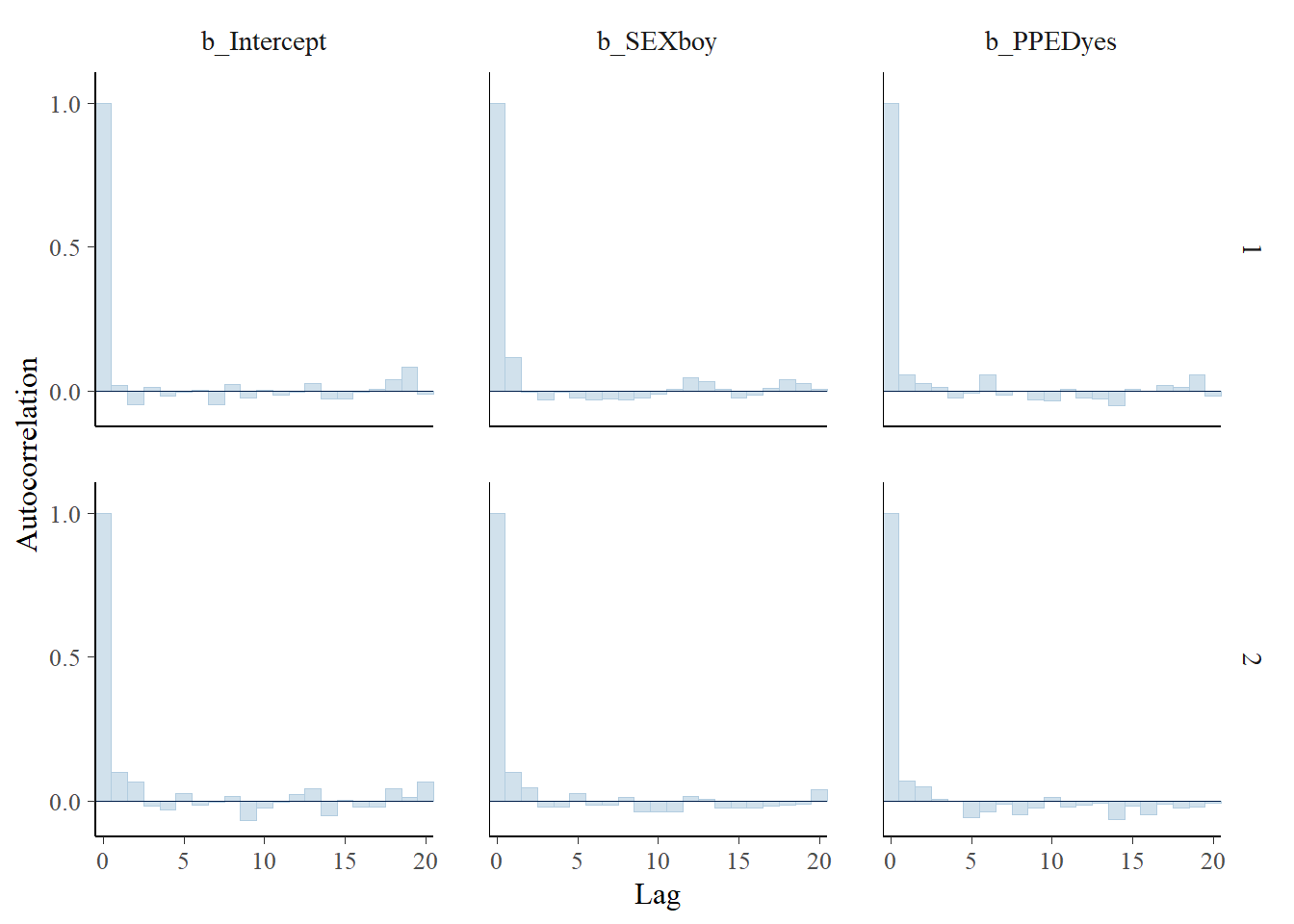The plot shows no evidence of autocorrelation for all model variables in both chains, as the autocorrelation parameters all quickly diminish to around zero.

#### 5.4. Interpretation

Now, we can safely proceed to the interpretation of the model. Below is the model summary of the Bayesian binary logistic regression model.

summary(Bayes_Model_Binary)
##  Family: bernoulli
## Formula: REPEAT ~ SEX + PPED
##    Data: ThaiEdu_New (Number of observations: 7516)
## Samples: 2 chains, each with iter = 2000; warmup = 500; thin = 1;
##          total post-warmup samples = 3000
##
## Population-Level Effects:
##           Estimate Est.Error l-95% CI u-95% CI Eff.Sample Rhat
## Intercept    -1.77      0.06    -1.88    -1.65       2621 1.00
## SEXboy        0.43      0.07     0.30     0.57       2470 1.00
## PPEDyes      -0.61      0.07    -0.74    -0.48       2451 1.00
##
## Samples were drawn using sampling(NUTS). For each parameter, Eff.Sample
## is a crude measure of effective sample size, and Rhat is the potential
## scale reduction factor on split chains (at convergence, Rhat = 1).

For comparison, below is the model summary of the frequentist binary logistic regression model.

Model_Binary <- glm(formula = REPEAT ~ SEX + PPED,
data = ThaiEdu_New)
summary(Model_Binary)
##
## Call:
## glm(formula = REPEAT ~ SEX + PPED, family = binomial(link = "logit"),
##     data = ThaiEdu_New)
##
## Deviance Residuals:
##     Min       1Q   Median       3Q      Max
## -0.6844  -0.5630  -0.5170  -0.4218   2.2199
##
## Coefficients:
##             Estimate Std. Error z value Pr(>|z|)
## (Intercept) -1.76195    0.05798 -30.387  < 2e-16 ***
## SEXboy       0.42983    0.06760   6.358 2.04e-10 ***
## PPEDyes     -0.61298    0.06833  -8.971  < 2e-16 ***
## ---
## Signif. codes:  0 '***' 0.001 '**' 0.01 '*' 0.05 '.' 0.1 ' ' 1
##
## (Dispersion parameter for binomial family taken to be 1)
##
##     Null deviance: 6140.8  on 7515  degrees of freedom
## Residual deviance: 6016.2  on 7513  degrees of freedom
## AIC: 6022.2
##
## Number of Fisher Scoring iterations: 4

From the model summary above, we can see that the Bayesian model estimates are almost identical to those of the frequentist model. The interpretation of these estimates are the same in both frequentist and Bayesian models. Nevertheless, note that the interpretation of the uncertainty intervals is not the same between the two models. In the frequentist model, the idea behind using a 95% uncertainty interval (confidence interval) is that, under repeated sampling, 95% of the resulting uncertainy intervals would cover the true population value. That allows us to say that, for a given 95% confidence interval, we are 95% confident that this confidence interval contains the true population value. However, it does not allow us to say that there is a 95% chance that the confidence interval contains the true population value (i.e. frequentist uncertainty intervals are not probability statements). In contrast, in the Bayesian model, the 95% uncertainty interval (called credibility interval), which is more interpretable, states that there is 95% chance that the true population value falls within this interval. When the 95% credibility intervals do not contain zero, we conclude that the respective model parameters are likely meaningful.

Let’s visualise the point estimates and their associated uncertainty intervals, using the stanplot function.

stanplot(Bayes_Model_Binary,
type = "areas",
prob = 0.95)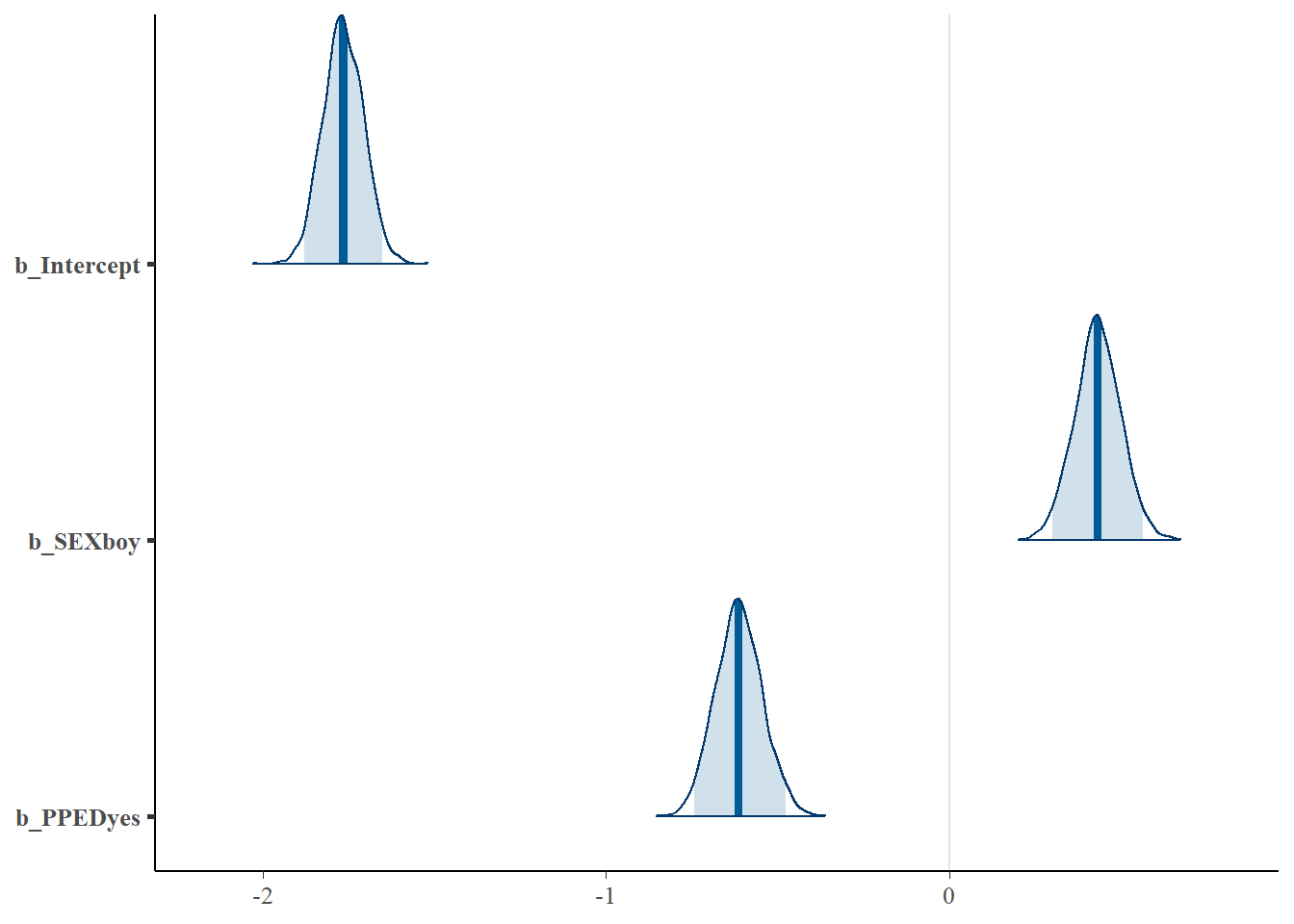The plot above shows the densities of the parameter estimates. The dark blue line in each density represents the point estimate, while the light-blue area indicates the 95% credibility intervals. We can easily see that both SEX and PPED are meaningful predictors, as their credibility intervals do not contain zero and their densities have a very narrow shape. SEX positively predicts a pupil’s probability of repeating a grade, while PPED negatively so. Specifically, in comparison to being a girl, being a boy is more likely to repeat a grade, assuming everything else stays constant. Having previous schooling is less likely to result in repeating a grade, assuming everything else stays constant.

To interpret the value of the parameter estimates, we need to exponentiate the estimates. See below.

exp(fixef(Bayes_Model_Binary)[,-2])
##            Estimate      Q2.5     Q97.5
## Intercept 0.1711235 0.1529269 0.1917295
## SEXboy    1.5411834 1.3490342 1.7598020
## PPEDyes   0.5418504 0.4755864 0.6217185

We can also plot densities of these parameter estimates. For this, we again use the stanplot function from brms.

stanplot(Bayes_Model_Binary,
type = "areas",
prob = 0.95,
transformations = "exp") +
geom_vline(xintercept = 1, color = "grey")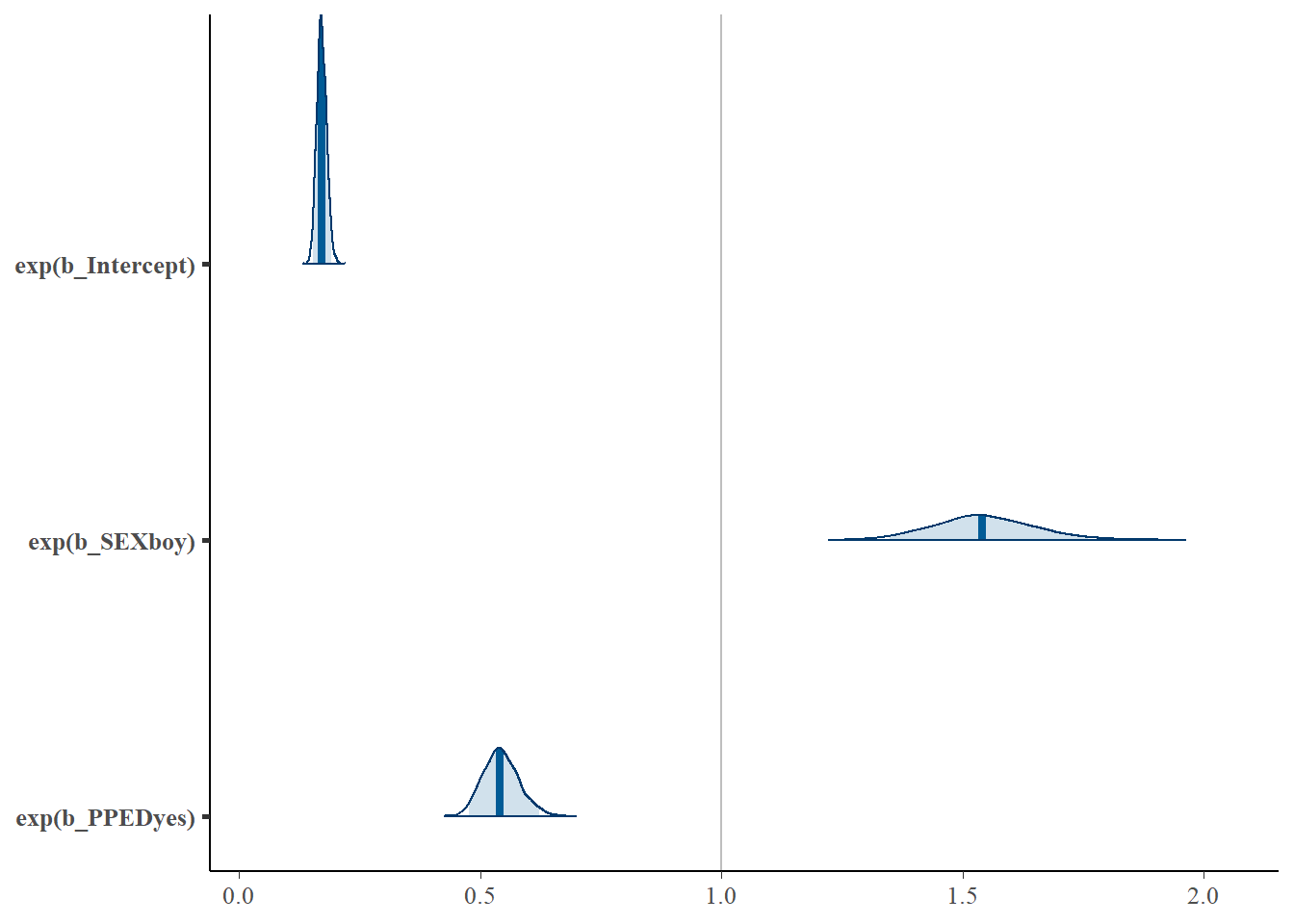Note that the interpretation of the parameter estimates is linked to the odds rather than probabilities. The definition of odds is: P(event occurring)/P(event not occurring). In this analysis, assuming everything else stays the same, being a boy increases the odds of repeating a grade by 54%, in comparison to being a girl; having preschool education lowers the odds of repeating a grade by (1 – 0.54)% = 46%, in comparison to not having preschool education, assuming everything else stays constant. The baseline odds (indicated by the intercept term) of repeating a grade, namely if you’re a girl with no previous schooling, is about 17%.

#### 5.4. Visualisation of Parameter Effects

We can plot the marginal effects (i.e. estimated probabilities of repeating a grade) of the variables in the model. Below, we show how different combinations of SEX and PPED result in different probability estimates. The advantage of this approach is that probabilities are more interpretable than odds.

ThaiEdu_New %>%
data_grid(SEX, PPED) %>%
ggplot(aes(x = .value, y = interaction(SEX, PPED))) +
stat_pointintervalh(.width = c(.68, .95)) +
coord_flip() +
xlab("predicted probability") +
scale_x_continuous(breaks = seq(0, 0.24, 0.02))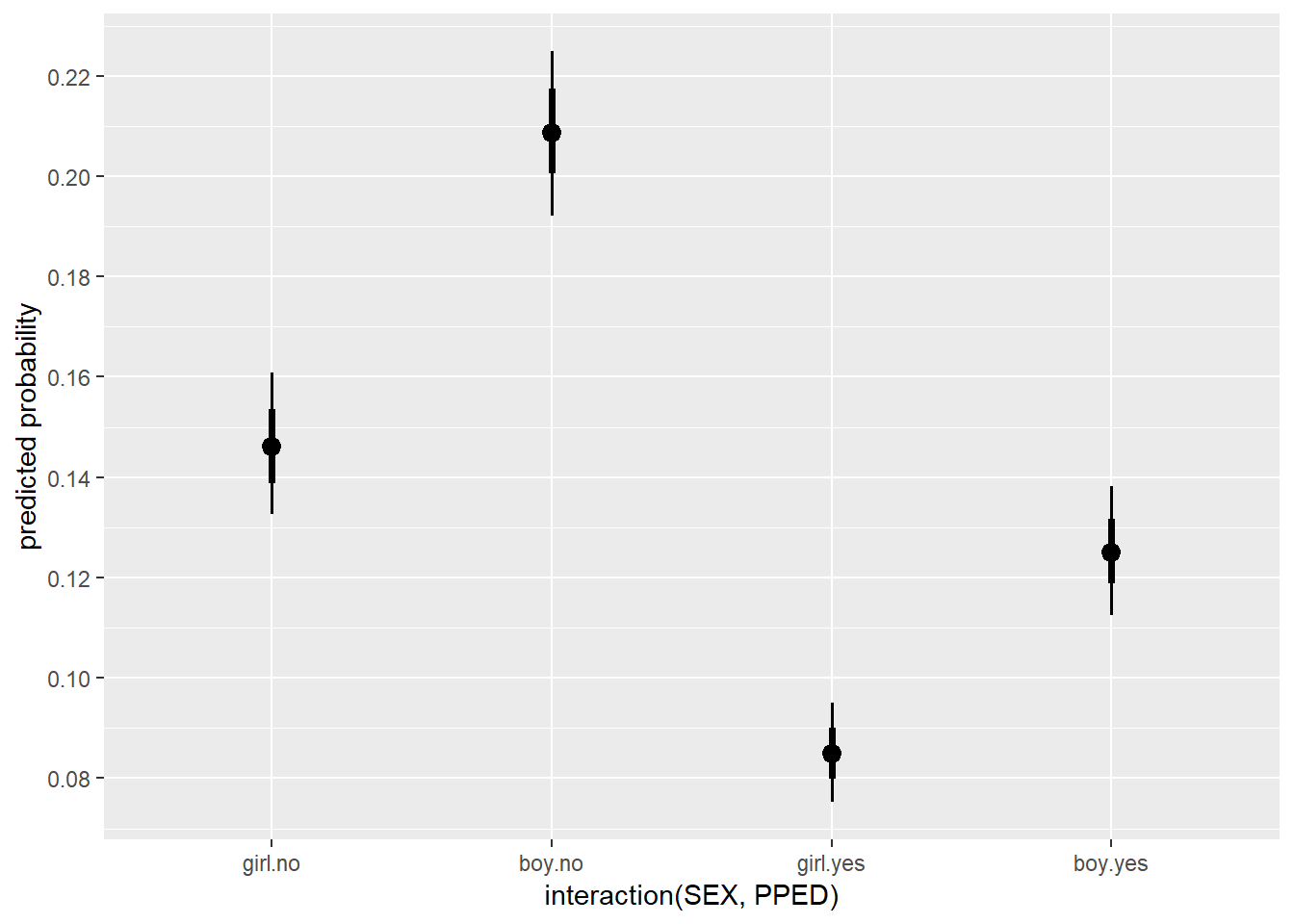As we can see, being a male pupil with no preschool education has the highest probability (~0.21), followed by being a girl with no preschool education (~0.15), being a boy with preschool education (~0.13), and lastly, being a girl with preschool education (~0.09). Note that both 68% (thicker inner lines) and 95% (thinner outer lines) credibility intervals for the estimates are included to give us some idea of the uncertainties of the estimates.

#### 5.5. Model Evaluation

In the Intro to Frequentist (Multilevel) Generalised Linear Models (GLM) in R with glm and lme4 tutorial, we learn that we can use the likelihood ratio test and AIC to assess the goodness of fit of the model(s). However, these two approaches do not apply to Bayesian models. Instead, Bayesian models make use of so-called Posterior Predictive P-values (PPPs) to assess the fit of the model. In addition, many also use Bayes factors to quantify support from the data for the model. This tutorial does not delve into PPPs or Bayes factors because of the complexity of the topics.

The other two measures mentioned in Intro to Frequentist (Multilevel) Generalised Linear Models (GLM) in R with glm and lme4 are correct classification rate and area under the curve (AUC). They are model-agnostic, meaning they can be applied to both frequentist and Bayesian models.

##### 5.5.1. Correct Classification Rate

The percentage of correct classification is a useful measure to see how well the model fits the data.

#use the predict() function to calculate the predicted probabilities of pupils in the original data from the fitted model
Pred <- predict(Bayes_Model_Binary, type = "response")
Pred <- if_else(Pred[,1] > 0.5, 1, 0)
ConfusionMatrix <- table(Pred, pull(ThaiEdu_New, REPEAT)) #pull results in a vector
#correct classification rate
sum(diag(ConfusionMatrix))/sum(ConfusionMatrix)
##  0.8580362
ConfusionMatrix
##
## Pred    0    1
##    0 6449 1067

We can see that the model correctly classifies 85.8% of all the observations. However, a closer look at the confusion matrix reveals that the model predicts all of the observations to belong to class “0”, meaning that all pupils are predicted not to repeat a grade. Given that the majority category of the REPEAT variable is 0 (No), the model does not perform better in classification than simply assigning all observations to the majority class 0 (No).

##### 5.5.2. AUC (area under the curve).

An alternative to using correct classification rate is the Area under the Curve (AUC) measure. The AUC measures discrimination, that is, the ability of the test to correctly classify those with and without the target response. In the current data, the target response is repeating a grade. We randomly pick one pupil from the “repeating a grade” group and one from the “not repeating a grade” group. The pupil with the higher predicted probability should be the one from the “repeating a grade” group. The AUC is the percentage of randomly drawn pairs for which this is true. This procedure sets AUC apart from the correct classification rate because the AUC is not dependent on the imblance of the proportions of classes in the outcome variable. A value of 0.50 means that the model does not classify better than chance. A good model should have an AUC score much higher than 0.50 (preferably higher than 0.80).

# Compute AUC for predicting Class with the model
Prob <- predict(Bayes_Model_Binary, type="response")
Prob <- Prob[,1]
Pred <- prediction(Prob, as.vector(pull(ThaiEdu_New, REPEAT)))
AUC <- performance(Pred, measure = "auc")
AUC <- AUC@y.values[]
AUC
##  0.6014733

With an AUC score of close to 0.60, the model does not discriminate well.

## 6. Bayesian Binomial Logistic Regression (with Non-Informative Priors)

As explained in the Intro to Frequentist (Multilevel) Generalised Linear Models (GLM) in R with glm and lme4 tutorial, logistic regression can also be used to model count or proportion data. Binary logistic regression assumes that the outcome variable comes from a bernoulli distribution (which is a special case of binomial distributions) where the number of trial $$n$$ is 1 and thus the outcome variable can only be 1 or 0. In contrast, binomial logistic regression assumes that the number of the target events follows a binomial distribution with $$n$$ trials and probability $$q$$. In this way, binomial logistic regression allows the outcome variable to take any non-negative integer value and thus is capable of handling count data.

The Thai Educational Data records information about individual pupils that are clustered within schools. By aggregating the number of pupils who repeated a grade by school, we obtain a new data set where each row represents a school, with information about the proportion of pupils repeating a grade in that school. The MSESC (mean SES score) is also on the school level; therefore, it can be used to predict proportion or count of pupils who repeat a grade in a particular school. See below.

#### 6.1. Tranform Data

ThaiEdu_Prop <- ThaiEdu_New %>%
group_by(SCHOOLID, MSESC) %>%
summarise(REPEAT = sum(REPEAT),
TOTAL = n()) %>%
ungroup()

head(ThaiEdu_Prop)
## # A tibble: 6 x 4
##   SCHOOLID MSESC REPEAT TOTAL
##   <fct>    <dbl>  <dbl> <int>
## 1 10103     0.88      1    17
## 2 10104     0.2       0    29
## 3 10105    -0.07      5    18
## 4 10106     0.47      0     5
## 5 10108     0.76      3    19
## 6 10109     1.06      9    21

In this new data set, REPEAT refers to the number of pupils who repeated a grade; TOTAL refers to the total number of students in a particular school.

#### 6.2. Explore data

ThaiEdu_Prop %>%
ggplot(aes(x = exp(MSESC)/(1+exp(MSESC)), y = REPEAT/TOTAL)) +
geom_point() +
geom_smooth(method = "lm")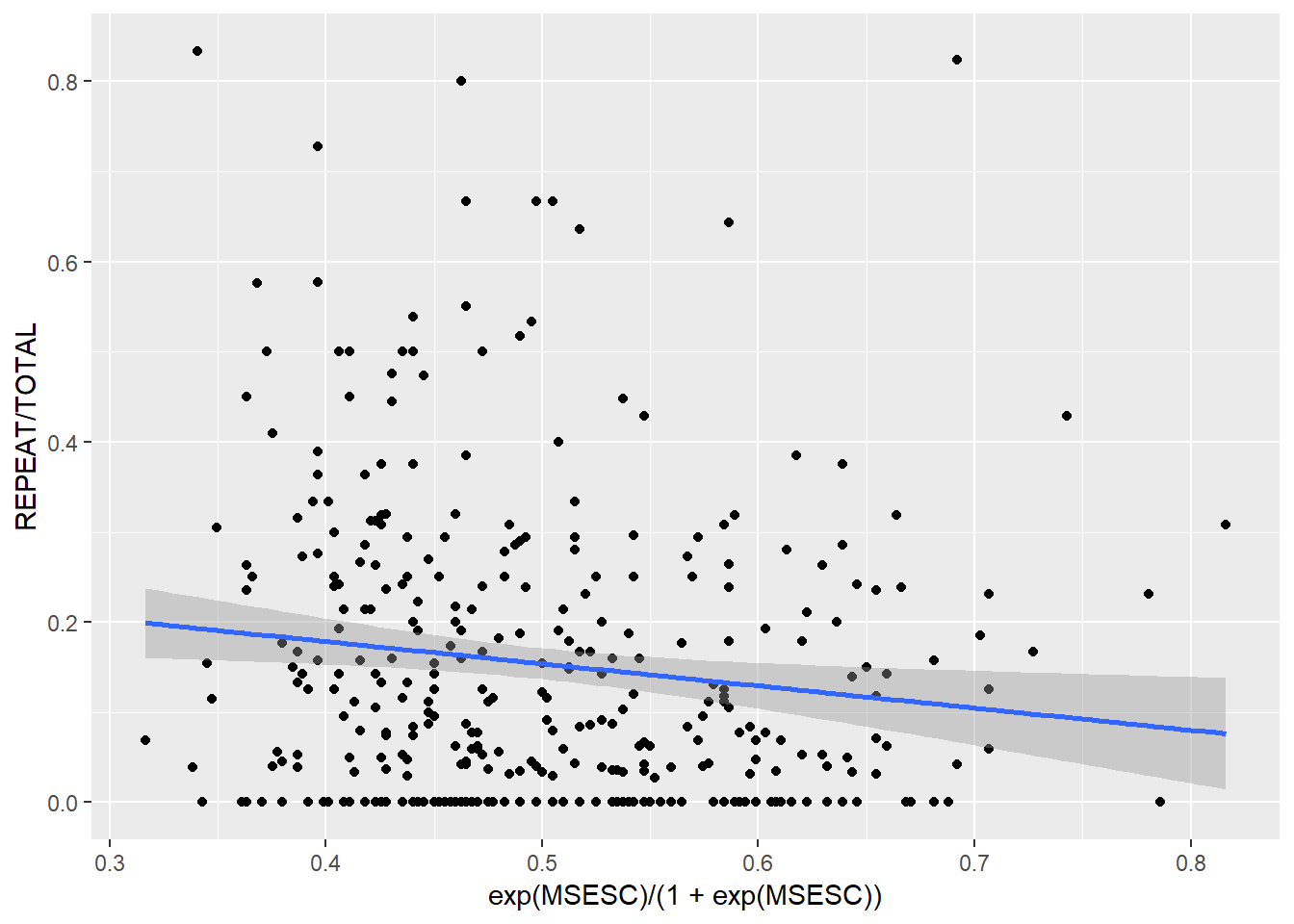We can see that the proportion of students who repeated a grade is (moderately) negatively related to the inverse-logit of MSESC. Note that we model the variable MSESC as its inverse-logit because in a binomial regression model, we assume a linear relationship between the inverse-logit of the linear predictor and the outcome (i.e. proportion of events), not linearity between the predictor itself and the outcome.

#### 6.3. Fit a Binomial Logistic Regression Model

To fit a Bayesian binomial logistic regression model, we also use the brm function like we did with the previous Bayesian binary logistic regression model. There are, however, two differences: First, to specify the outcome variable in the formula, we need to specify both the number of target events (REPEAT) and the total number of trials (TOTAL) wrapped in trials(), which are separated by |. In addition, the family should be “binomial” instead of “bernoulli”.

Bayes_Model_Prop <- brm(REPEAT | trials(TOTAL) ~ MSESC,
data = ThaiEdu_Prop,
warmup = 500,
iter = 2000,
chains = 2,
inits = "0",
cores = 2,
seed = 123)
## Compiling the C++ model
## Start sampling
summary(Bayes_Model_Prop)
##  Family: binomial
## Formula: REPEAT | trials(TOTAL) ~ MSESC
##    Data: ThaiEdu_Prop (Number of observations: 356)
## Samples: 2 chains, each with iter = 2000; warmup = 500; thin = 1;
##          total post-warmup samples = 3000
##
## Population-Level Effects:
##           Estimate Est.Error l-95% CI u-95% CI Eff.Sample Rhat
## Intercept    -1.81      0.03    -1.87    -1.74       2743 1.00
## MSESC        -0.44      0.09    -0.62    -0.26       2478 1.00
##
## Samples were drawn using sampling(NUTS). For each parameter, Eff.Sample
## is a crude measure of effective sample size, and Rhat is the potential
## scale reduction factor on split chains (at convergence, Rhat = 1).

The frequentist model (for comparison):

Model_Prop <- glm(formula = cbind(REPEAT, TOTAL-REPEAT) ~ MSESC,
family = binomial(logit),
data = ThaiEdu_Prop)

summary(Model_Prop)
##
## Call:
## glm(formula = cbind(REPEAT, TOTAL - REPEAT) ~ MSESC, family = binomial(logit),
##     data = ThaiEdu_Prop)
##
## Deviance Residuals:
##     Min       1Q   Median       3Q      Max
## -3.3629  -1.8935  -0.5083   1.1674   6.9494
##
## Coefficients:
##             Estimate Std. Error z value Pr(>|z|)
## (Intercept) -1.80434    0.03324 -54.280  < 2e-16 ***
## MSESC       -0.43644    0.09164  -4.763 1.91e-06 ***
## ---
## Signif. codes:  0 '***' 0.001 '**' 0.01 '*' 0.05 '.' 0.1 ' ' 1
##
## (Dispersion parameter for binomial family taken to be 1)
##
##     Null deviance: 1480.7  on 355  degrees of freedom
## Residual deviance: 1457.3  on 354  degrees of freedom
## AIC: 2192
##
## Number of Fisher Scoring iterations: 5

We can see that the model estimates between the Bayesian and the frequentist binomial logistic regression models are very similar. Note that we skipped the step of checking model convergence, for the sake of keeping this tutorial shorter. You can use the same codes we showed before (with the binary logistic regression model) to check the convergence of this model.

#### 6.4. Interpretation

The parameter interpretation in a binomial regression model is the same as that in a binary logistic regression model. We know from the model summary above that the mean SES score of a school is negatively related to the odds of students repeating a grade in that school. To enhance interpretability, we again calculate the exponentiated coefficient estimate of MSESC. Since MSESC is a continous variable, we can standardise the exponentiated MSESC estimate (by multiplying the original estimate with the SD of the variable, and then then exponentiating the resulting number).

exp(fixef(Bayes_Model_Prop)[2,-2]*sd(pull(ThaiEdu_Prop, MSESC), na.rm = T))
##  Estimate      Q2.5     Q97.5
## 0.8465065 0.7897812 0.9056835

We can see that with a SD increase in MSESC, the odds of students repeating a grade is lowered by about (1 – 85%) = 15%. “Q2.5” and “Q97.5” refer to the lower bound and the upper bound of the uncertainty interval, respectively. This credibility interval does not contain zero, suggesting that the variable is likely meaningful.

We can visualise the effect of MSESC.

Bayes_Model_Prop %>%
mutate(MSESC = list(seq(-0.77, 1.49, 0.01))) %>% #the observed value range of MSESC
unnest(MSESC) %>%
mutate(pred = exp(b_Intercept + b_MSESC*MSESC)/(1+exp(b_Intercept + b_MSESC*MSESC))) %>%
group_by(MSESC) %>%
summarise(pred_m = mean(pred, na.rm = TRUE),
pred_low = quantile(pred, prob = 0.025),
pred_high = quantile(pred, prob = 0.975)) %>%
ggplot(aes(x = MSESC, y = pred_m)) +
geom_line() +
geom_ribbon(aes(ymin = pred_low, ymax = pred_high), alpha=0.2) +
ylab("Predicted Probability of Repeating a Grade") +
scale_y_continuous(breaks = seq(0, 0.22, 0.01))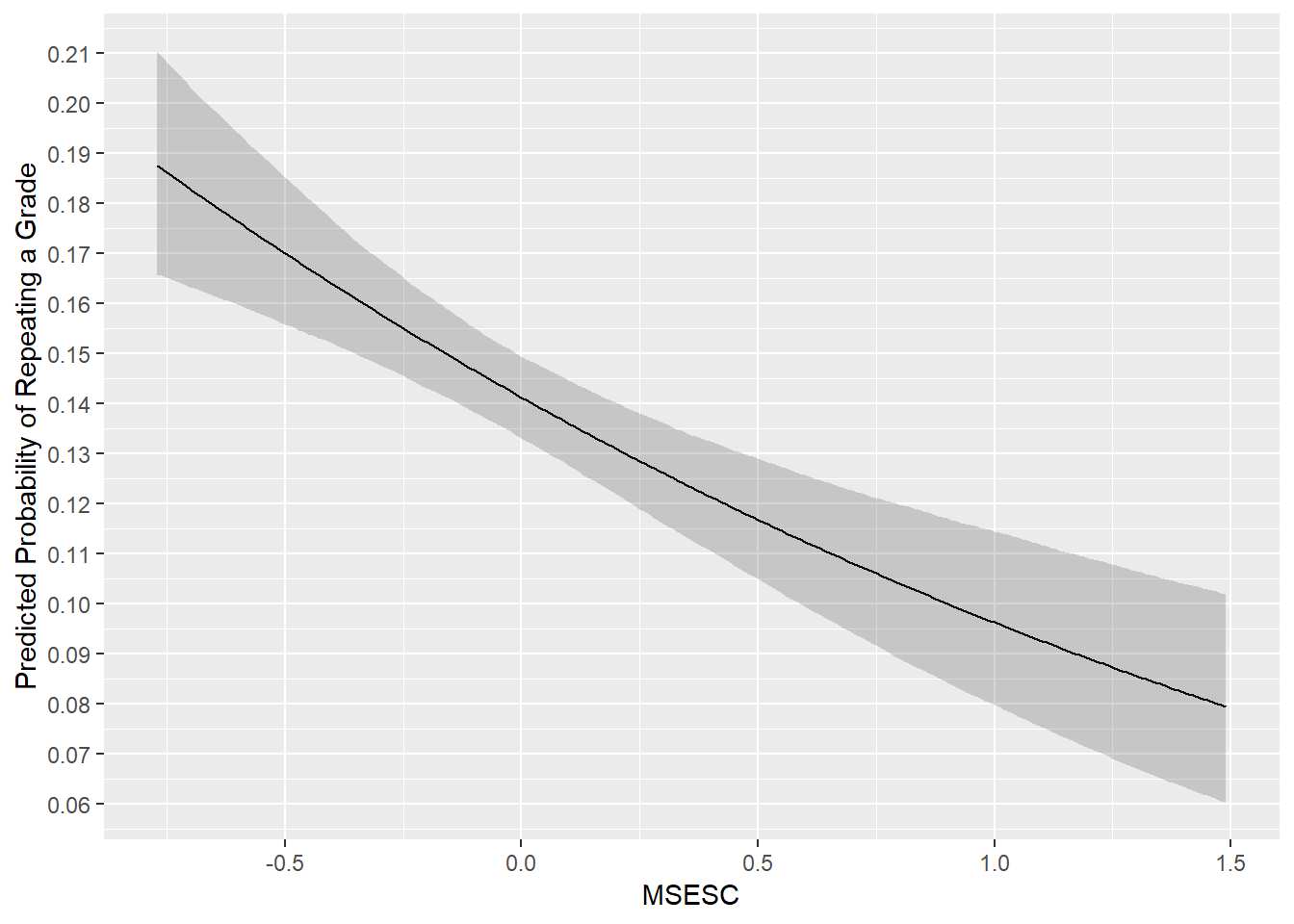The plot above shows the expected influence of MSESC on the probability of a pupil repeating a grade. Holding everything else constant, as MSESC increases, the probability of a pupil repeating a grade lowers (from 0.19 to 0.08). The grey shaded areas indicate the 95% credibility intervals of the predicted values at each value of MSESC.

#### 6.5. Model Evaluation

Similar to the Bayesian binary logistic regression model, we can use the PPPS and Bayes factor (which are not discussed in this tutorial) to evaluate the fit of a Bayesian binomial logistic regression model. Correct classification rate and AUC are not suited here, as the model is not concerned with classification.

## 7. Bayesian Multilevel Binary Logistic Regression (with Non-Informative Priors)

The Bayesian binary logistic regression model introduced earlier is limited to modelling the effects of pupil-level predictors; the Bayesian binomial logistic regression is limited to modelling the effects of school-level predictors. To incorporate both pupil-level and school-level predictors, we can use multilevel models, specifically, Bayesian multilevel binary logistic regression. If you are unfamiliar with multilevel models, you can use Multilevel analysis: Techniques and applications for reference and this tutorial for a good introduction to multilevel models with the lme4 package in R.

In addition to the motivation above, there are more reasons to use multilevel models. For instance, as the data are clustered within schools, it is likely that pupils from the same school are more similar to each other than those from other schools. Because of this, in one school, the probability of a pupil repeating a grade may be high, while in another school, low. Furthermore, even the relationship between the outcome (i.e. repeating a grade) and the predictor variabales (e.g. gender, preschool education, SES) may be different across schools. Also note that there are missing values in the MSESC variable. Using multilevel models can appropriately address these issues.

See the following plot as an example. The plot shows the proportions of students repeating a grade across schools. We can see vast differences across schools. Therefore, we need multilevel models.

ThaiEdu_New %>%
group_by(SCHOOLID) %>%
summarise(PROP = sum(REPEAT)/n()) %>%
plot()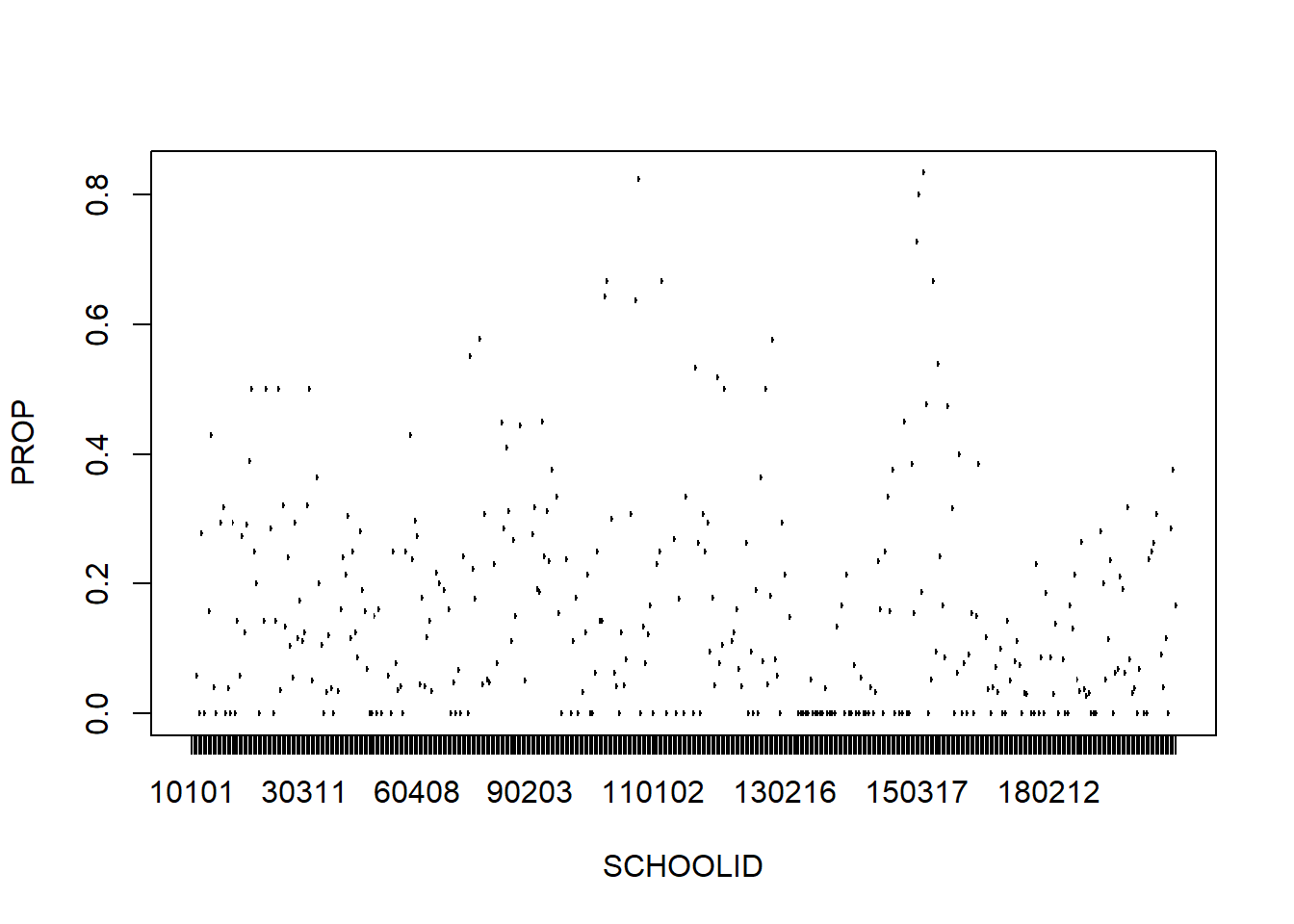We can also plot the relationship between SEX and REPEAT by SCHOOLID, to see whether the relationship between gender and repeating a grade differs by school.

ThaiEdu_New %>%
mutate(SEX = if_else(SEX == "boy", 1, 0)) %>%
ggplot(aes(x = SEX, y = REPEAT, color = as.factor(SCHOOLID))) +
geom_point(alpha = .1, position = "jitter")+
geom_smooth(method = "glm", se = F,
method.args = list(family = "binomial")) +
theme(legend.position = "none") +
scale_x_continuous(breaks = c(0, 1)) +
scale_y_continuous(breaks = c(0, 1))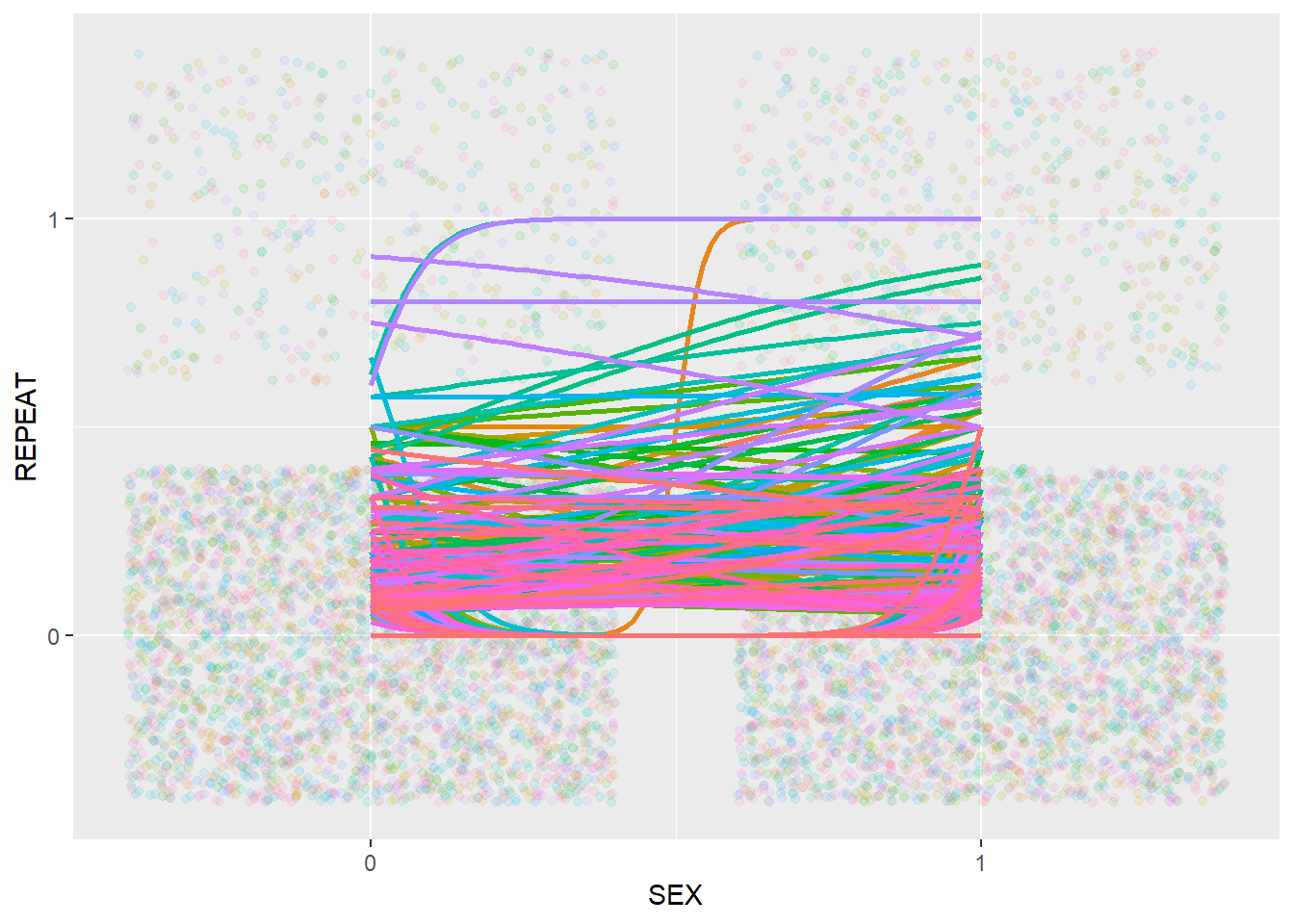In the plot above, different colors represent different schools. We can see that the relationship between SEX and REPEAT appears to be quite different across schools.

We can make the same plot for PPED and REPEAT.

ThaiEdu_New %>%
mutate(PPED = if_else(PPED == "yes", 1, 0)) %>%
ggplot(aes(x = PPED, y = REPEAT, color = as.factor(SCHOOLID))) +
geom_point(alpha = .1, position = "jitter")+
geom_smooth(method = "glm", se = F,
method.args = list(family = "binomial")) +
theme(legend.position = "none") +
scale_x_continuous(breaks = c(0, 1)) +
scale_y_continuous(breaks = c(0, 1))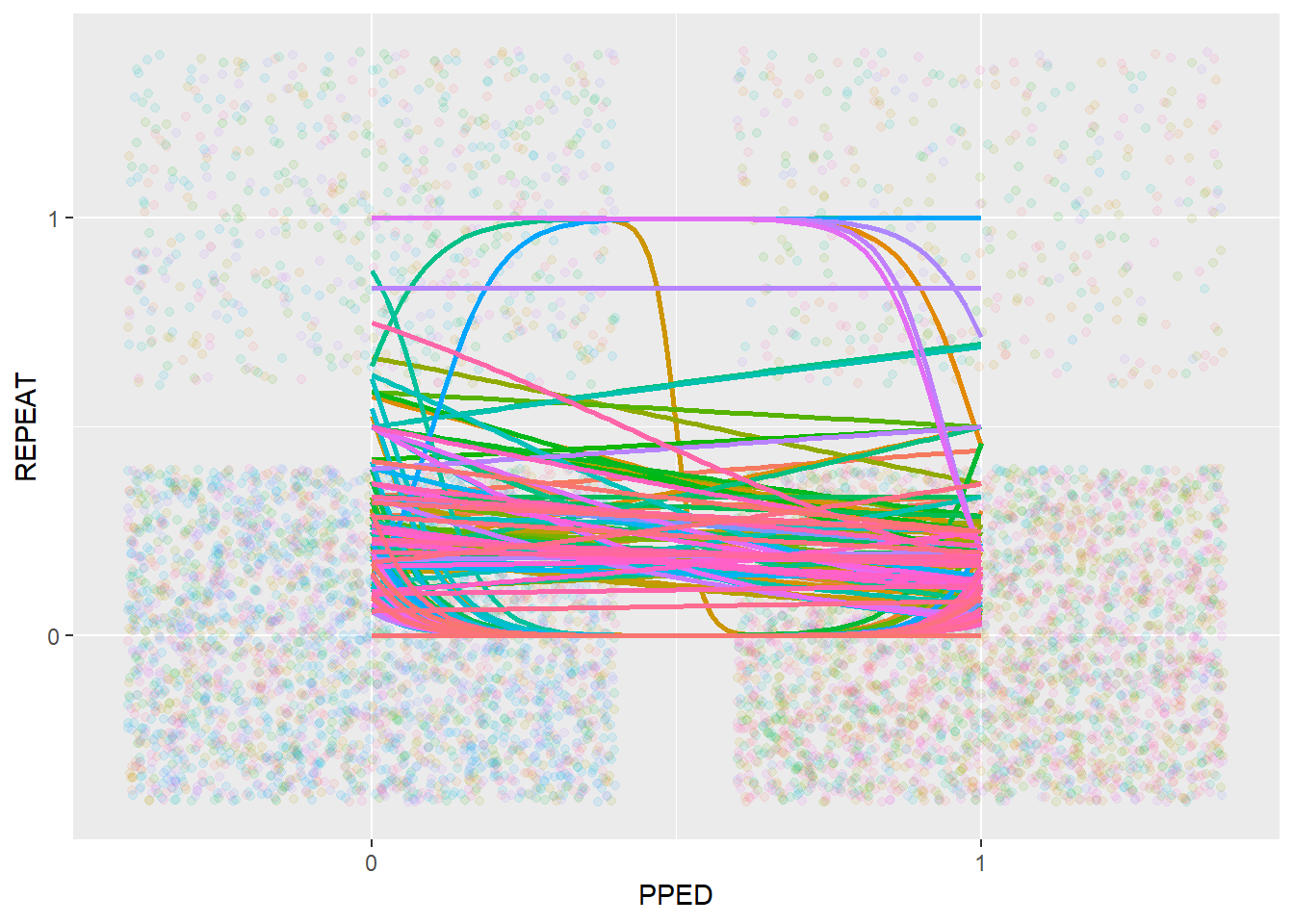The relationship between PPED and REPEAT also appears to be quite different across schools. However, we can also see that most of the relationships follow a downward trend, going from 0 (no previous schooling) to 1 (with previous schooling), indicating a negative relationship between PPED and REPEAT.

Because of the observations above, we can conclude that there is a need for multilevel modelling in the current data, with not only a random intercept (SCHOOLID) but potentially also random slopes of the SEX and PPED.

#### 7.1. Center Variables

Prior to fitting a multilevel model, it is necessary to center the predictors by using an appropriately chosen centering method (i.e. grand-mean centering or within-cluster centering), because the centering approach matters for the interpretation of the model estimates. Following the advice of Enders and Tofighi (2007), we should use within-cluster centering for the first-level predictors SEX and PPED, and grand-mean centering for the second-level predictor MSESC.

ThaiEdu_Center <- ThaiEdu_New %>%
mutate(SEX = if_else(SEX == "girl", 0, 1),
PPED = if_else(PPED == "yes", 1, 0)) %>%
group_by(SCHOOLID) %>%
mutate(SEX = SEX - mean(SEX),
PPED = PPED - mean(PPED)) %>%
ungroup() %>%
mutate(MSESC = MSESC - mean(MSESC, na.rm = T))

head(ThaiEdu_Center)
## # A tibble: 6 x 5
##   SCHOOLID    SEX   PPED    REPEAT MSESC
##   <fct>     <dbl>  <dbl> <dbl+lbl> <dbl>
## 1 10103    -0.647 -0.882    0 [no] 0.870
## 2 10103    -0.647 -0.882    0 [no] 0.870
## 3 10103    -0.647  0.118    0 [no] 0.870
## 4 10103    -0.647  0.118    0 [no] 0.870
## 5 10103    -0.647  0.118    0 [no] 0.870
## 6 10103    -0.647  0.118    0 [no] 0.870

#### 7.2. Intercept Only Model

To specify a multilevel model, we again use the brm function from the brms package. Note that the random effect term should be included in parentheses. In addition, within the parentheses, the random slope term(s) and the cluster terms should be separated by |.

We start by specifying an intercept-only model, in order to assess the impact of the clustering structure of the data. Note that we will skip the step of model convergence diagnostics.

Bayes_Model_Multi_Intercept <- brm(REPEAT ~ 1 + (1|SCHOOLID),
data = ThaiEdu_Center,
warmup = 500,
iter = 2000,
chains = 2,
inits = "0",
cores = 2,
seed = 123)
## Compiling the C++ model
## Start sampling

Below we calculate the ICC (intra-class correlation) of the intercept-only model. Note that for non-Gaussian Bayesian models (e.g. logistic regression), we need to set “ppd = T” such that the variance calculation is based on the posterior predictive distribution.

icc(Bayes_Model_Multi_Intercept, ppd = T)
##
## # Random Effect Variances and ICC
##
## Family: bernoulli (logit)
## Conditioned on: all random effects
##
## ## Variance Ratio (comparable to ICC)
## Ratio: 0.29  HDI 89%: [0.20 0.37]
##
## ## Variances of Posterior Predicted Distribution
## Conditioned on fixed effects: 0.09  HDI 89%: [0.08 0.10]
## Conditioned on rand. effects: 0.12  HDI 89%: [0.12 0.13]
##
## ## Difference in Variances
## Difference: 0.03  HDI 89%: [0.02 0.05]

A variance ratio (comparable to ICC) of 0.29 means that 29% of the variation in the outcome variable can be accounted for by the clustering stucture of the data. This provides evidence that a multilevel model may make a difference to the model estimates, in comparison with a non-multilevel model. Therefore, the use of multilevel models is necessary and warrantied.

#### 7.3. Full Model

It is good practice to build a multilevel model step by step. However, as this tutorial’s focus is not on muitilevel modelling, we go directly from the intercept-only model to the full-model that we are ultimately interested in. In the full model, we include not only fixed effect terms of SEX, PPED and MSESC and a random intercept term, but also random slope terms for SEX and PPED. Note that we specify family = bernoulli(link = "logit"), as this model is essentially a binary logistic regression model.

Bayes_Model_Multi_Full <- brm(REPEAT ~ SEX + PPED + MSESC + (1 + SEX + PPED|SCHOOLID),
data = ThaiEdu_Center,
warmup = 500,
iter = 2000,
chains = 2,
inits = "0",
cores = 2,
seed = 123)
## Compiling the C++ model
## Start sampling
summary(Bayes_Model_Multi_Full)
##  Family: bernoulli
## Formula: REPEAT ~ SEX + PPED + MSESC + (1 + SEX + PPED | SCHOOLID)
##    Data: ThaiEdu_Center (Number of observations: 7516)
## Samples: 2 chains, each with iter = 2000; warmup = 500; thin = 1;
##          total post-warmup samples = 3000
##
## Group-Level Effects:
## ~SCHOOLID (Number of levels: 356)
##                     Estimate Est.Error l-95% CI u-95% CI Eff.Sample Rhat
## sd(Intercept)           1.34      0.08     1.19     1.50        851 1.00
## sd(SEX)                 0.38      0.18     0.05     0.73        492 1.00
## sd(PPED)                0.26      0.18     0.01     0.69        800 1.00
## cor(Intercept,SEX)      0.42      0.29    -0.22     0.93       1298 1.00
## cor(Intercept,PPED)    -0.20      0.43    -0.90     0.75       2392 1.00
## cor(SEX,PPED)           0.01      0.49    -0.86     0.87       1502 1.00
##
## Population-Level Effects:
##           Estimate Est.Error l-95% CI u-95% CI Eff.Sample Rhat
## Intercept    -2.29      0.09    -2.47    -2.12        801 1.00
## SEX           0.45      0.11     0.22     0.66       1615 1.00
## PPED         -0.60      0.13    -0.86    -0.34       2826 1.00
## MSESC        -0.51      0.22    -0.94    -0.07        743 1.00
##
## Samples were drawn using sampling(NUTS). For each parameter, Eff.Sample
## is a crude measure of effective sample size, and Rhat is the potential
## scale reduction factor on split chains (at convergence, Rhat = 1).

We can plot the densities of the relevant model parameter estimates.

stanplot(Bayes_Model_Multi_Full,
type = "areas",
prob = 0.95)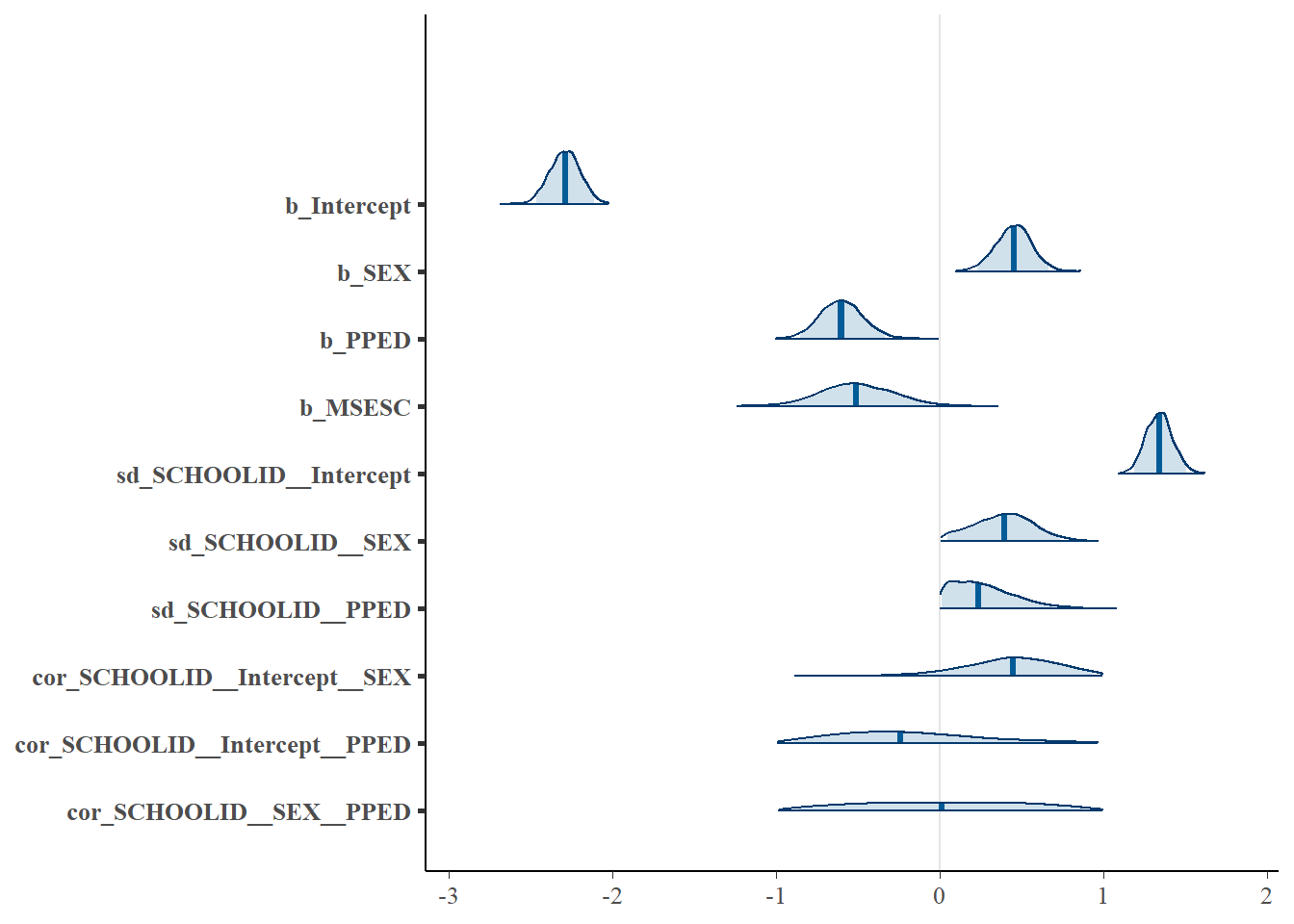The results (pertaining to the fixed effects) are similar to the results of the previous Bayesian binary logistic regression and binomial logistic regression models. On the pupil-level, SEX has a positive influence on the odds of a pupil repeating a grade, while PPED has a negative influence. On the school-level, MSESC has a negative effect on the outcome variable. Among three predictors, SEX and PPED have credibility intervals (indicated by the shaded light blue regions in the densities) that clearly do not contain zero. Therefore, they should be treated as meaningful predictors. In contrast, MSESC, despite having a 95% credibility interval without zero, the upper bound of the credibility interval is very close to zero, and its density only contains zero. Because of this, MSESC is likely a less relevant predictor than SEX and PPED.

Now let’s look at the random effect terms (sd(Intercept), sd(SEX) and sd(PPED)). The density of sd(Intercept) in the plot is clearly away from zero, indicating the relevance of including this random intercept term in the model. The variance of the random slope of SEX is $$0.38^2 = 0.14$$, and that of PPED is $$0.26^2 = 0.07$$. Both variances are not negligible. However, if we look at the density plot, the lower bounds of the credibility intervals of both sd(SEX) and sd(PPED) are very close to zero, and their densities also not clearly separate from zero. This suggests that including these two random slope terms may not be necessary.

We can also plot the random effect terms across schools.

#extract posterior distributions of all the random effect terms
data_RandomEffect <- ranef(Bayes_Model_Multi_Full)

#extract posterior distributions of sd(Intercept)
r_Intercept <- data_RandomEffect$SCHOOLID[, , 1] %>% as_tibble() %>% rownames_to_column(var = "SCHOOLID") %>% mutate(Variable = "sd(Intercept)") #extract posterior distributions of sd(SEX) r_SEX <- data_RandomEffect$SCHOOLID[, , 2] %>%
as_tibble() %>%
rownames_to_column(var = "SCHOOLID") %>%
mutate(Variable = "sd(SEX)")

#extract posterior distributions of sd(PPED)
r_PPED <- data_RandomEffect\$SCHOOLID[, , 3] %>%
as_tibble() %>%
rownames_to_column(var = "SCHOOLID") %>%
mutate(Variable = "sd(PPED)")

#plot
r_Intercept %>%
bind_rows(r_SEX) %>%
bind_rows(r_PPED) %>%
mutate(Contain_Zero = if_else(Q2.5*Q97.5 > 0, "no", "yes")) %>%
ggplot(aes(x = SCHOOLID, y = Estimate, col = Contain_Zero)) +
geom_point() +
geom_errorbar(aes(ymin=Q2.5, ymax=Q97.5)) +
facet_grid(. ~ Variable, scale = "free") +
coord_flip() +
theme(legend.position = "top")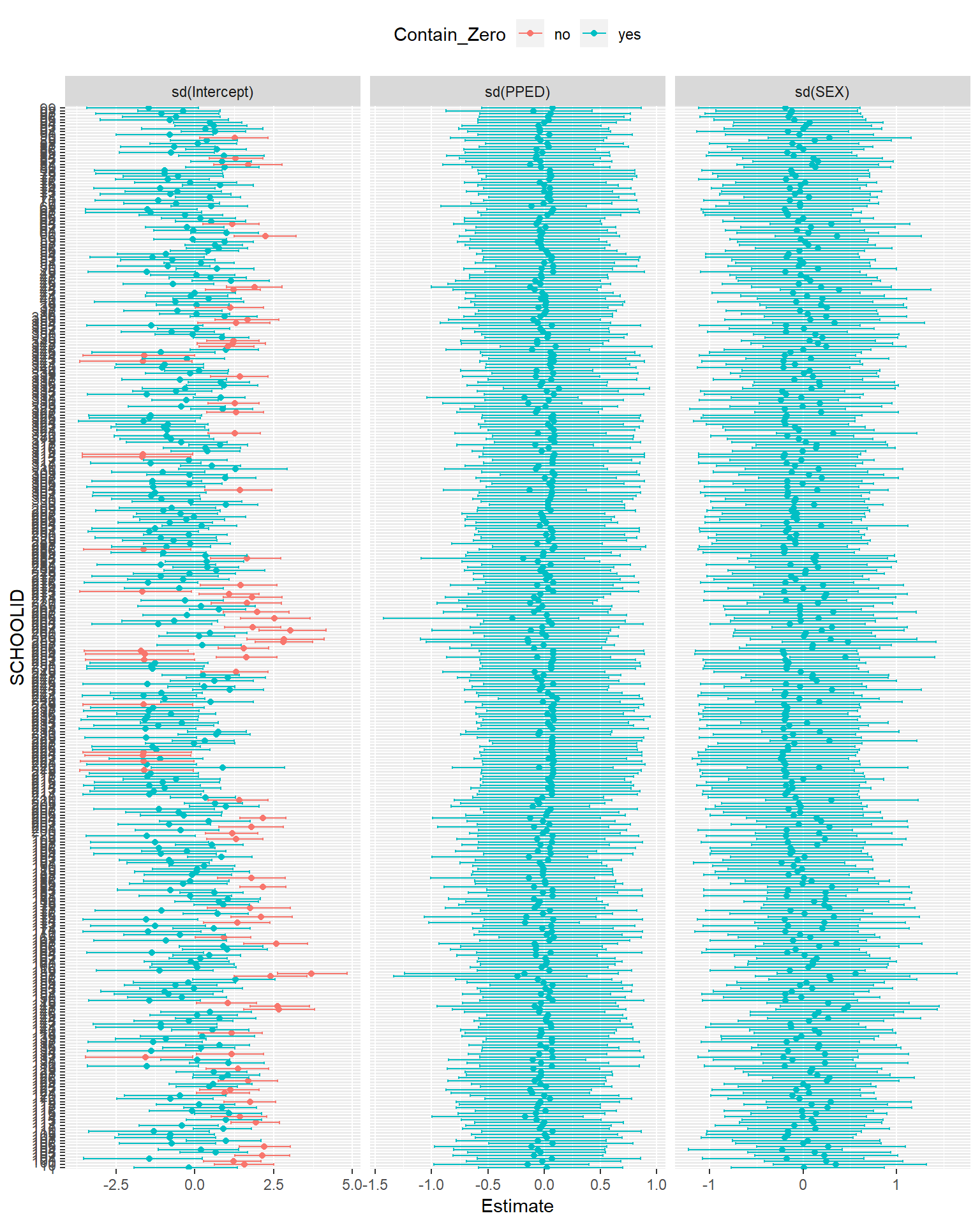Again, we can see that the posterior distributions of the random intercept term (sd(Intercept)) have a large variance across schools. Quite a number of them are also away from zero. Therefore, we can conclude that the inclusion of the random intercept is necessary. In comparison, all of the posterior distributions of sd(SEX) and sd(PPED) go through zero, suggesting that there is probably no need to include the two random slopes in the model.

To interpret the fixed-effect terms, we can calculate the exponentiated coefficient estimates.

#the categorical variables: SEX and PPED
exp(fixef(Bayes_Model_Multi_Full)[-4,-2])
##            Estimate      Q2.5     Q97.5
## Intercept 0.1010137 0.0846112 0.1205179
## SEX       1.5655171 1.2513685 1.9344052
## PPED      0.5473489 0.4244672 0.7135445
#the continous variable: MSESC
exp(fixef(Bayes_Model_Multi_Full)[4,-2]*sd(pull(ThaiEdu_Prop, MSESC), na.rm = T))
##  Estimate      Q2.5     Q97.5
## 0.8237781 0.6998895 0.9747519

We can see that the effects of SEX, PPED, and MSESC are very similar to the prevoius model results.